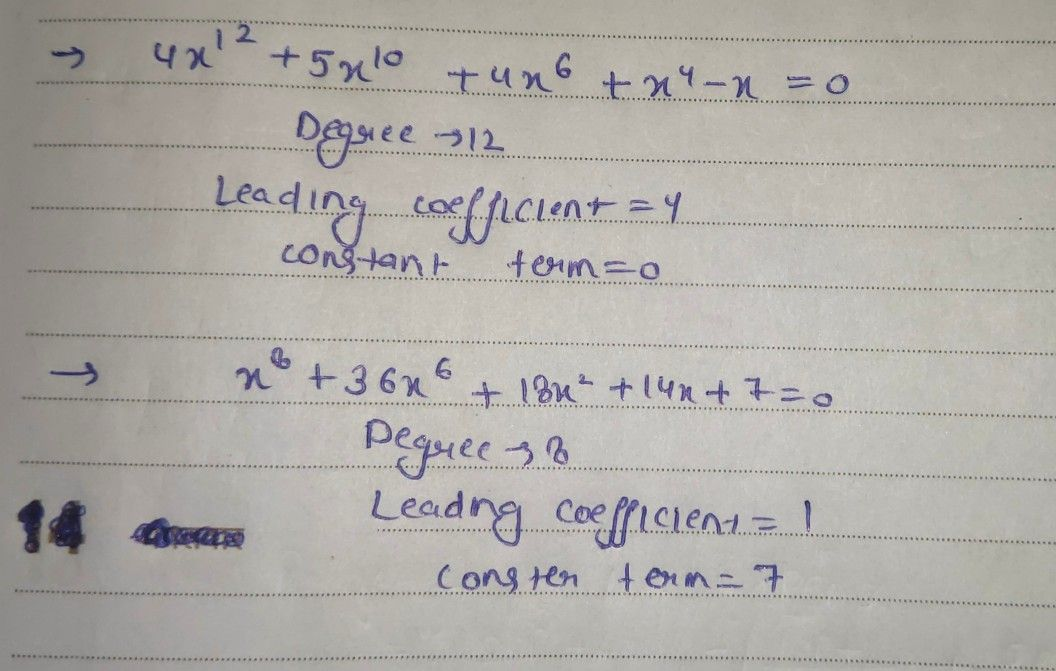Symbol
ProblemB. Write the terms of expression in the descending order. Determine the degree, the leading coefficient and the $const0n$ term. Polynomial Descending Order Degree Leading $constsn1$ Equations $co$ Coefficient Term $4.5x^{20}+4x^{12}+$ $4x^{6}+x^{4}-x=0$ $5.x^{3}+14x+36x^{6}$ $+18x^{2}+7=0$ $6.3x^{4}-16x^{2}+$ $21x^{3}+4x+12=0$ the table helow hy identifving the degree and real roots of